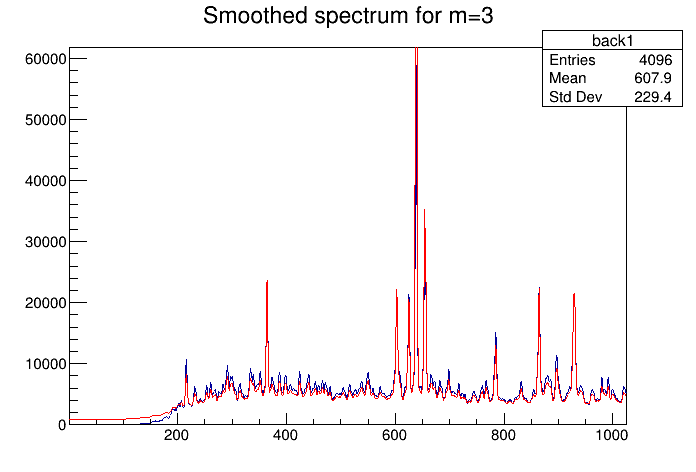# Smoothing¶

Example to illustrate smoothing using Markov algorithm (class TSpectrum).

Author: Miroslav Morhac
This notebook tutorial was automatically generated with ROOTBOOK-izer from the macro found in the ROOT repository on Sunday, October 02, 2022 at 09:38 AM.

In :
Int_t i;
const Int_t nbins = 1024;
Double_t xmin  = 0;
Double_t xmax  = nbins;
Double_t source[nbins];
gROOT->ForceStyle();

TString dir  = gROOT->GetTutorialDir();
TString file = dir+"/spectrum/TSpectrum.root";
TFile *f     = new TFile(file.Data());
TH1F *h = (TH1F*) f->Get("back1");
h->SetTitle("Smoothed spectrum for m=3");

for (i = 0; i < nbins; i++) source[i]=h->GetBinContent(i + 1);
h->SetAxisRange(1,1024);
h->Draw("L");

TSpectrum *s = new TSpectrum();

TH1F *smooth = new TH1F("smooth","smooth",nbins,0.,nbins);
smooth->SetLineColor(kRed);

s->SmoothMarkov(source,1024,3);  //3, 7, 10
for (i = 0; i < nbins; i++) smooth->SetBinContent(i + 1,source[i]);
smooth->Draw("L SAME");

Info in <TCanvas::MakeDefCanvas>:  created default TCanvas with name c1


Draw all canvases

In :
gROOT->GetListOfCanvases()->Draw()